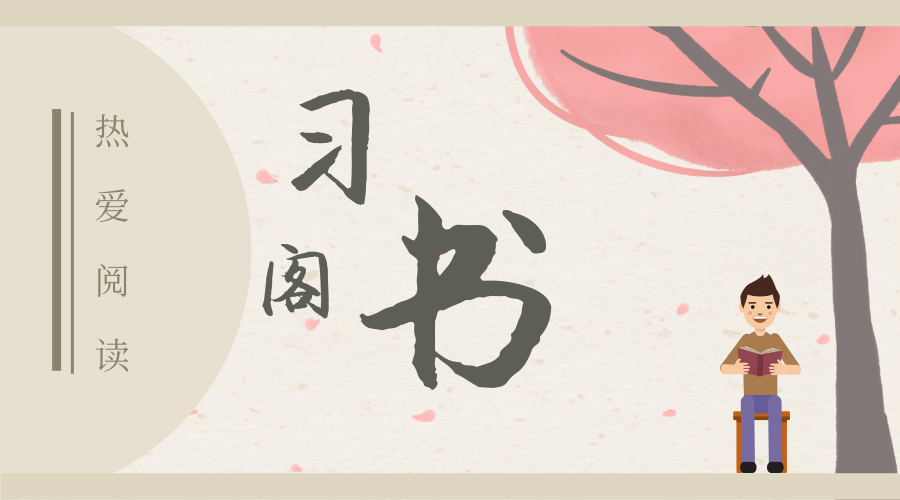# 机器语言、汇编语言、高级语言都包含什么

0+0=0，0+1=1，1+0=1，1+1=10 ，0×0=0，0×1=0，1×0=0，1×1=1

1+9=10，2+8=10，3+7=10，4+6=10，5+5=10，6+4=10，7+3=10，8+2=10，9+1=10…….请登录后查看回复内容